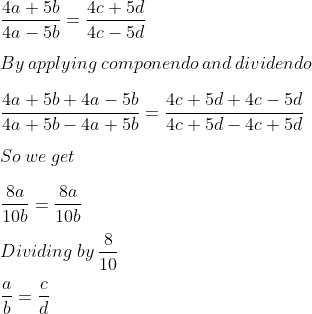Guru

# Question 3. If (4a + 5b) (4c – 5d) = (4a – 5d) (4c + 5d), prove that a, b, c, d are in proporton.

• 0

This is a basic question from ML aggarwal book of class 10th, chapter 7, ratio and proportion., ICSE board

In this question we have been given that

(4a + 5b) (4c – 5d) = (4a – 5d) (4c + 5d),

And we have to apply mathematical operations to prove that a, b, c, d are in proporton.

Question no 3, 7.3

Share

1. Solution:

It is given that

(4a + 5b) (4c – 5d) = (4a – 5d) (4c + 5d)

We can write it asTherefore, it is proved that a, b, c, d are in proportion.

• 0